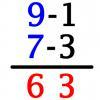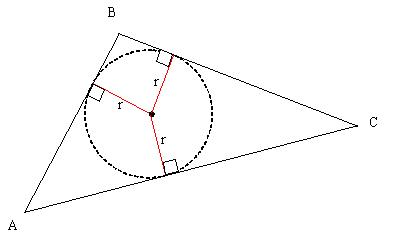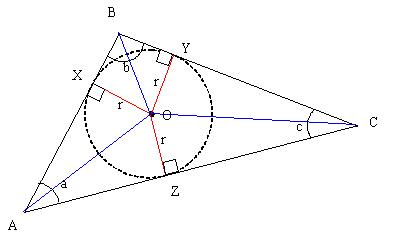#### You may also like### Vedic Sutra - All from 9 and Last from 10

Vedic Sutra is one of many ancient Indian sutras which involves a cross subtraction method. Can you give a good explanation of WHY it works?### Tournament Scheduling

Scheduling games is a little more challenging than one might desire. Here are some tournament formats that sport schedulers use.### Archimedes and Numerical Roots

The problem is how did Archimedes calculate the lengths of the sides of the polygons which needed him to be able to calculate square roots?

# Triangle Incircle Iteration

##### Age 14 to 16Challenge Level

Meg offers this solution:

The tangent of a circle is at right-angles to the radius of the circle. That is, if you join the centre point of the circle to a point where the circle meets the outer triangle, it makes an angle of $90 ^{\circ}$ with the side of the triangle.The bisector of the angles of triangle $ABC$ will all pass through the centre of the circle.From this we know that
$OAZ = \frac {a}{2}$ and $OZA = 90 ^{\circ}$
Hence $AOZ = 90- \frac{a}{2}$
Now consider triangle XOZ. This triangle is isosceles, so $OXZ = XZO = \frac{180-(180-a)}{2} = \frac{a}{2}$

By similar arguments
$OXY = OYZ = \frac{b}{2}$ and $OYZ = OZY = \frac{c}{2}$ Hence the new angles of the triangle are

$ZXY= \frac{a}{2}+\frac{b}{2}$

$XYZ = \frac{b}{2} + \frac{c}{2}$

$YZX =\frac{c}{2} + \frac{a}{2}$

$a+b+c=180$

Hence $\frac{a+b}{2}= 90 - \frac{c}{2}$

It follows that $ZXY =90 - \frac {c}{2}$.

A similar argument can be followed for $XYZ$ and $YZX$. If you continue drawing triangles within circles, the angles will decrease as shown here:

Triangle 1: a
Triangle 2: $90 - \frac{a}{2}$
Triangle 3: $90- \frac{90-\frac{a}{2}}{2}$ =$90 - \frac{90}{2} + \frac{a}{4}$
Triangle 4: $90- \frac{90-\frac{90}{2}+\frac{a}{4}}{2}= \frac{3}{4}.90 - \frac{a}{8}$

When you continue this iteration, you can demonstrate that the $a$ term becomes less and less significant, and the sum of the rest of the terms tends to 60 degrees. Hence the triangle tends to an equilateral triangle.